Courses

# Stereochemistry & Asymmetric Synthesis

## 20 Questions MCQ Test GATE Chemistry Mock Test Series | Stereochemistry & Asymmetric Synthesis

Description
This mock test of Stereochemistry & Asymmetric Synthesis for Chemistry helps you for every Chemistry entrance exam. This contains 20 Multiple Choice Questions for Chemistry Stereochemistry & Asymmetric Synthesis (mcq) to study with solutions a complete question bank. The solved questions answers in this Stereochemistry & Asymmetric Synthesis quiz give you a good mix of easy questions and tough questions. Chemistry students definitely take this Stereochemistry & Asymmetric Synthesis exercise for a better result in the exam. You can find other Stereochemistry & Asymmetric Synthesis extra questions, long questions & short questions for Chemistry on EduRev as well by searching above.
QUESTION: 1

### The stereochemistry of this molecule is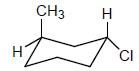Solution: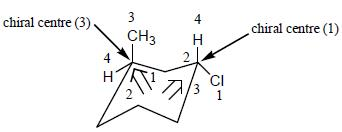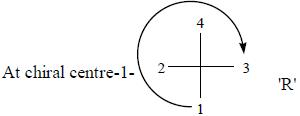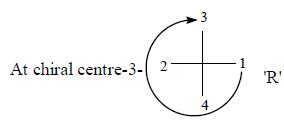QUESTION: 2

###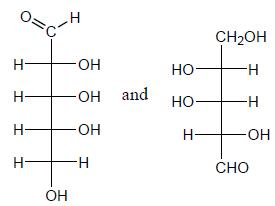The above pair is the case of

Solution: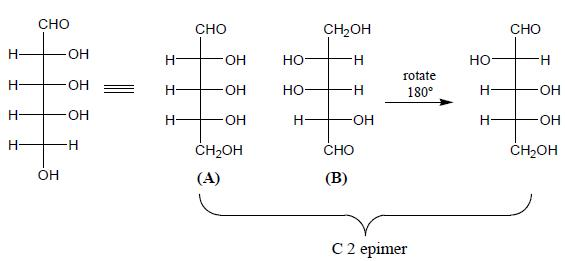QUESTION: 3

### Amongest the following the correct statement for the compound P, Q and R is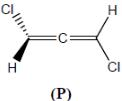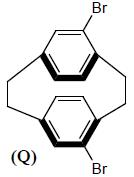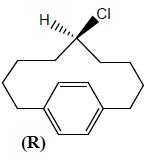Solution: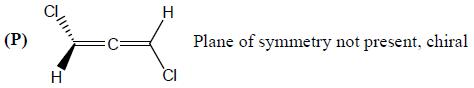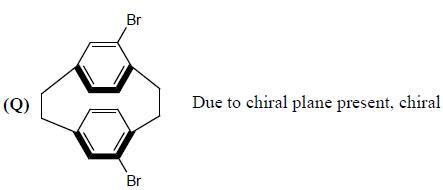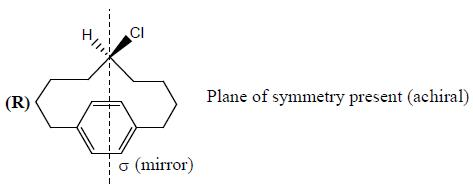QUESTION: 4

Among the following, the optically inactive compounds is/are

Solution: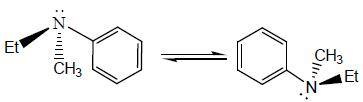Due to pyramidal inversion, optically inactives.

*Answer can only contain numeric values
QUESTION: 5

Vivacept used in the treatment of HIN and mestranol is an oral contraceptive. The total number of asymmetric centre in viracept are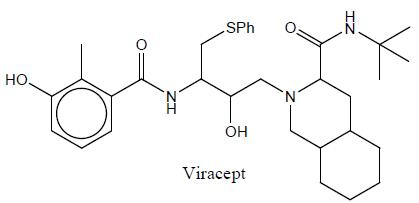Solution: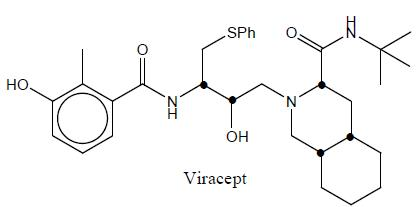Note : (•) Asymmetric centre

QUESTION: 6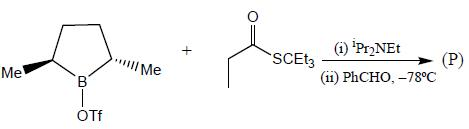Solution:

Csymmetric dialkyl boron with bulky thiopropionate ester followed by addition of an aldehyde result in the anti-aldol product with high optical purity.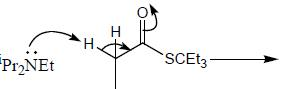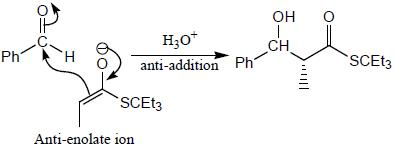QUESTION: 7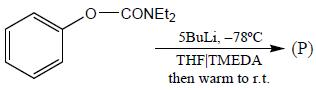Solution: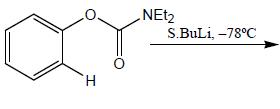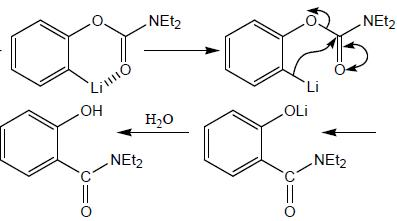QUESTION: 8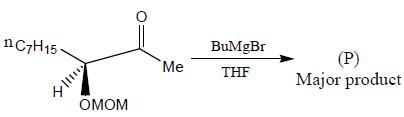Solution: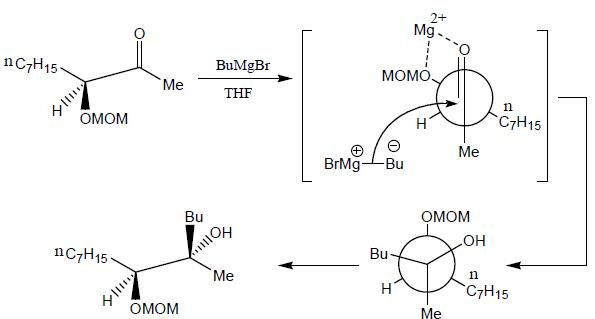QUESTION: 9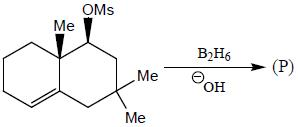Solution: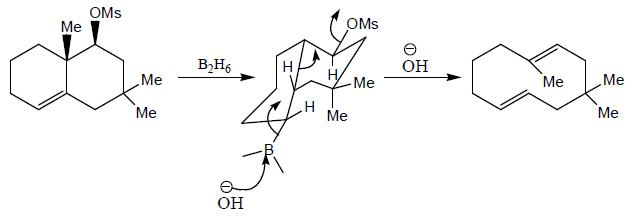QUESTION: 10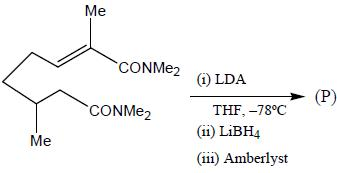Solution: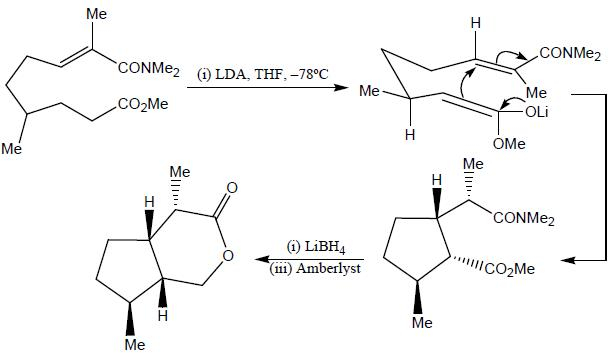QUESTION: 11

The absolute configurations for compounds X and Y respectively are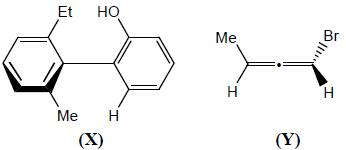Solution: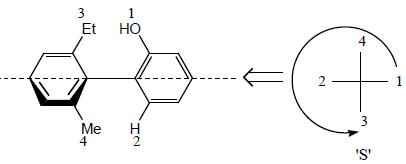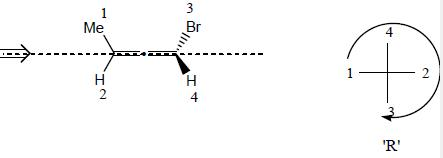QUESTION: 12

Which two of the following compounds represents a pair of enantiomers.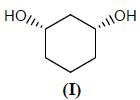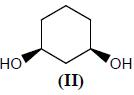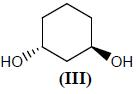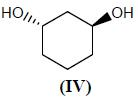Solution: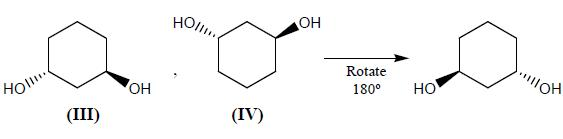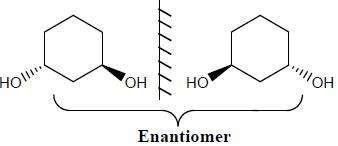QUESTION: 13

The configuration (R/S notation) at C-1 and C-6 of the compound below areSolution: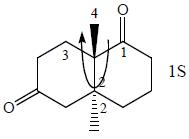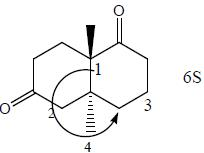QUESTION: 14

Consider the given structure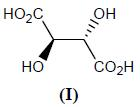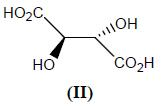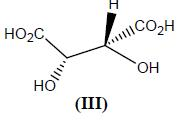Solution: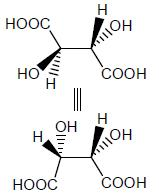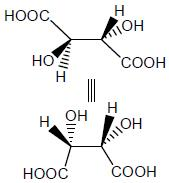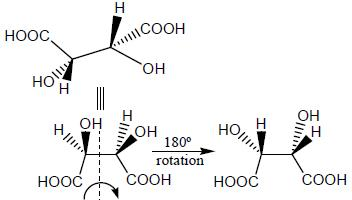QUESTION: 15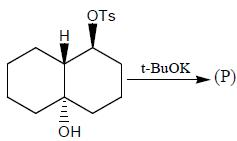Solution: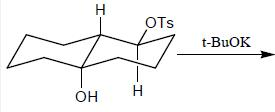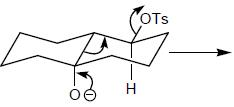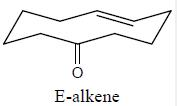QUESTION: 16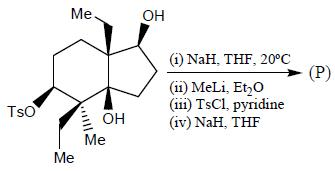Solution: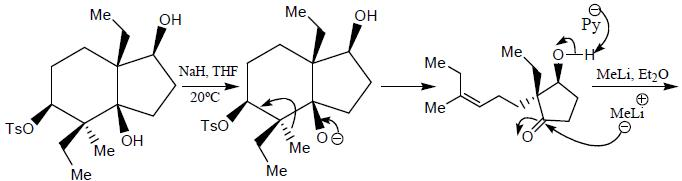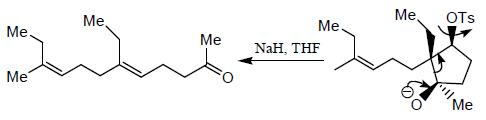QUESTION: 17

The major product formed in the following reaction sequence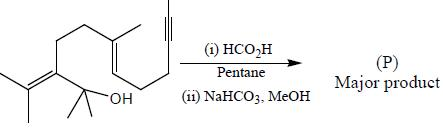Solution: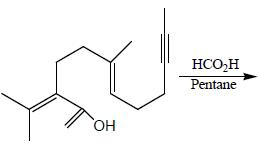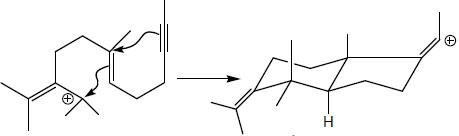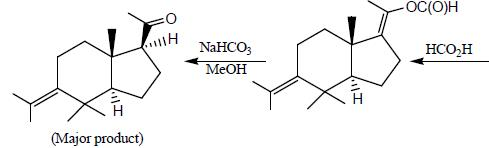QUESTION: 18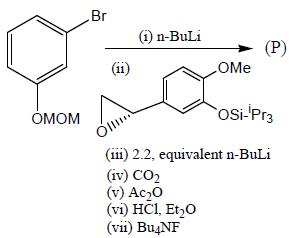Solution: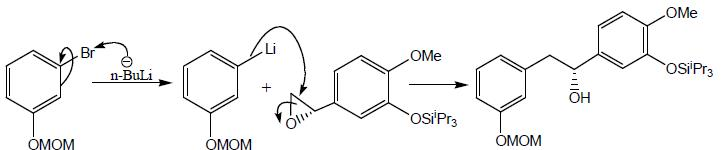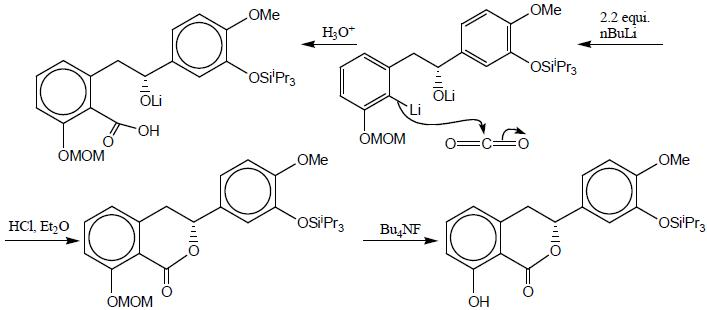*Answer can only contain numeric values
QUESTION: 19

In the following how many structures (Hydrogens marked Ha and Hb) are enantiotopic.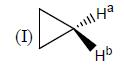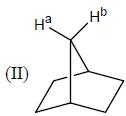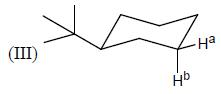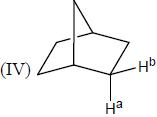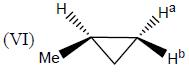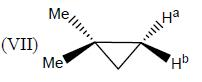Solution: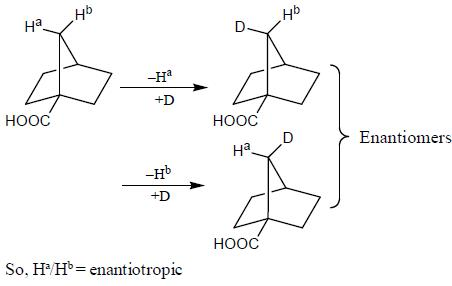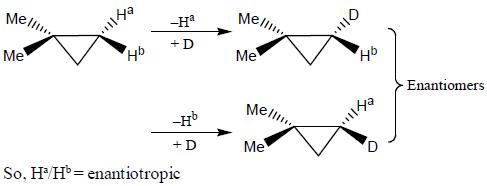*Answer can only contain numeric values
QUESTION: 20

How many in the following compounds is/are chiral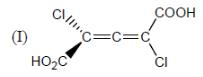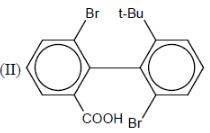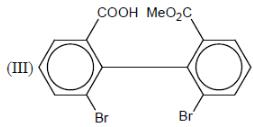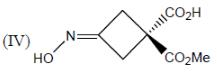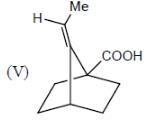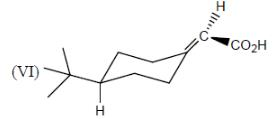Solution:

In case,
(I) Due to presence of chiral axis.
(II) In this case, both pheyl ring in different plane
(III) Both phenyl ring in different plane. Chiral plane are present
(IV) due to chiral plane is present
(V) no any symmetry are present
(VI) Chiral compound